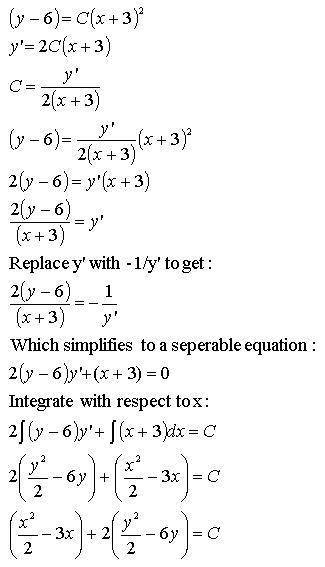# Family of orthogonal trajectories for a vertical parabola

• DaConfusion
In summary, the procedure for finding the family of orthogonal trajectories includes determining the differential equation for the given family, replacing y' in the equation, and finding the general solution. Additionally, when completing the square, it may be simpler to integrate the equation in a different way.

#### DaConfusion

1. Homework Statement . The correct answer is E2. Homework Equations :Procedure from our text:

"Step 1. Determine the differential equation for the given family F(x, y,C) = 0.
Step 2. Replace y' in that equation by −1/y'; the resulting equation is the differential equation
for the family of orthogonal trajectories.
Step 3. Find the general solution of the new differential equation. This is the family of orthogonal
trajectories."

## The Attempt at a SolutionThank you all for looking/helping!

You might want to start by reversing x and y in you original equation: if a parabola has vertical axis and vertex at (3, 6) then it is of the form
y= C(x-3)2+ 6. Also, looking at the way the possible solutions are given, I would NOT multiply out the squares.

Oh...that vertical was confusing me like crazy! I kept thinking the axis is parallel to the asymptote not orthogonal. Thanks.I still do not see it. Has to be some simple mistake...At one point, you have
$$2(y-6)= y'(x+3)$$
(You have, accidently, "-" instead of "=")
but the next line is
$$\frac{2(y-6)}{x-3}= y'$$
where it obviously should be "x+3" instead of "x-3".

$$\frac{x^2}{2}+3x+ y^2- 12y= C$$
(Notice that I have changed your "-3x" to "+3x")
Complete the square:
that's the same as
$$x^2+ 6x+ 2(y^2- 12y) = 2C$$
$$x^2+ 6x+ 9+ 2(y^2- 12y+ 36)= 2C+ 9+ 72$$
$$= (x+ 3)^2+ 2(y- 6)^2= 2C+9+72= C'$$
which is one of your options.

By the way, it would be simpler to integrate
$$2\int (y-6)dy+ \int (x+3)dx= 0$$
as
[tex](y-6)^2+ \frac{1}{2}(x+3)^2= C[/itex]
This differs from your integral only by the constant C.

perfect!

Thanks for the help I can see it now.